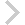FICO Xpress Optimization Examples Repository
 FICO Optimization Community FICO Xpress Optimization HomeGoal programming

Description
This example tries to construct a production plan which meets four different goals in order of priority.

Source Files
By clicking on a file name, a preview is opened at the bottom of this page.

GoalProg.cs

/***********************************************************************
Goal programming example
========================

An example of lexicographic goal programming using the Xpress
multi-objective API.

A company produces two electrical products, A and B. Both require
two stages of production: wiring and assembly. The production plan
must meet several goals:
1. A profit of $200 2. A contractual requirement of 40 units of product B 3. To fully utilize the available wiring department hours 4. To avoid overtime in the assembly department (c) 2022-2023 Fair Isaac Corporation ***********************************************************************/ using System; using Optimizer; namespace XPRSExamples { class GoalProg { private static readonly int COLS = 8; // Number of columns private static readonly int ROWS = 4; // Number of rows private static readonly int ENTITIES = 2; // Number of entities // Column indices private static readonly int COL_PRODUCE_A = 0; private static readonly int COL_PRODUCE_B = 1; private static readonly int COL_SURPLUS_WIRING = 2; private static readonly int COL_DEFICIT_WIRING = 3; private static readonly int COL_SURPLUS_ASSEM = 4; private static readonly int COL_DEFICIT_ASSEM = 5; private static readonly int COL_DEFICIT_PROFIT = 6; private static readonly int COL_DEFICIT_PROD_B = 7; public static void Main(string[] args) { GoalProg example = new GoalProg(); example.Run(); } private void Run() { try { XPRS.Init(""); prob = new XPRSprob(); // Tell Optimizer to call OptimizerMsg whenever a message is output prob.AddMessageCallback(new MessageCallback(this.OptimizerMsg)); // Create two integer variables and six continuous variables double[] ub = new double; char[] coltype = new char; int[] colind = new int; for (int i = 0; i < 8; i++) { ub[i] = XPRS.PLUSINFINITY; coltype[i] = i < 2 ? 'I' : 'C'; colind[i] = i; } prob.AddCols(8, 0, new double, new int, null, null, new double, ub); prob.ChgColType(8, colind, coltype); // Add one row per goal int ncoef = 0; char[] rowtype = new char; double[] rhs = new double; int[] start = new int; double[] rowcoef = new double; // Goal 1: profit must meet or exceed$200
colind[ncoef] = COL_PRODUCE_A; rowcoef[ncoef++] = 7;
colind[ncoef] = COL_PRODUCE_B; rowcoef[ncoef++] = 6;
colind[ncoef] = COL_DEFICIT_PROFIT; rowcoef[ncoef++] = 1;
rowtype = 'G'; rhs = 200;
// Goal 2: production of product B must meet or exceed 40 units
start = ncoef;
colind[ncoef] = COL_PRODUCE_B; rowcoef[ncoef++] = 1;
colind[ncoef] = COL_DEFICIT_PROD_B; rowcoef[ncoef++] = 1;
rowtype = 'G'; rhs = 40;
// Goal 3: hours of wiring should be close to the available 120 hours
start = ncoef;
colind[ncoef] = COL_PRODUCE_A; rowcoef[ncoef++] = 2;
colind[ncoef] = COL_PRODUCE_B; rowcoef[ncoef++] = 3;
colind[ncoef] = COL_SURPLUS_WIRING; rowcoef[ncoef++] = -1;
colind[ncoef] = COL_DEFICIT_WIRING; rowcoef[ncoef++] = 1;
rowtype = 'E'; rhs = 120;
// Goal 4: hours of assembly should be close to the available 300 hours
start = ncoef;
colind[ncoef] = COL_PRODUCE_A; rowcoef[ncoef++] = 6;
colind[ncoef] = COL_PRODUCE_B; rowcoef[ncoef++] = 5;
colind[ncoef] = COL_SURPLUS_ASSEM; rowcoef[ncoef++] = -1;
colind[ncoef] = COL_DEFICIT_ASSEM; rowcoef[ncoef++] = 1;
rowtype = 'E'; rhs = 300;
prob.AddRows(4, ncoef, rowtype, rhs, start, colind, rowcoef);

// Define objectives to minimize deviations, in priority order
// Goal 1: minimize profit deficit
prob.ChgObj(1, new int[] { COL_DEFICIT_PROFIT }, new double[] { 1 });
// Goal 2: minimize production deficit
prob.ChgObjN(1, 1, new int[] { COL_DEFICIT_PROD_B }, new double[] { 1 });
// Goal 3: minimize deviation from wiring hours target
prob.ChgObjN(2, 2, new int[] { COL_SURPLUS_WIRING, COL_DEFICIT_WIRING }, new double[] { 1, 1 });
// Goal 4: minimize deviation from assembly hours target
prob.ChgObjN(3, 2, new int[] { COL_SURPLUS_ASSEM, COL_DEFICIT_ASSEM }, new double[] { 1, 1 });

// Set up objective priorities and tolerances
for (int i = 0; i < 4; i++)
{
prob.SetObjIntControl(i, ObjControl.Priority, 4 - i);
prob.SetObjDblControl(i, ObjControl.AbsTol, 0);
prob.SetObjDblControl(i, ObjControl.RelTol, 0);
}

// Solve the problem
prob.MipOptimize();

// Print the result
if (prob.SolveStatus == SolveStatus.Completed &&
prob.SolStatus == SolStatus.Optimal)
{
double[] sol = new double[COLS];
prob.GetMipSol(sol, null);
Console.WriteLine("Production plan:");
Console.WriteLine("Product A: " + ((int)sol[COL_PRODUCE_A]) + " units");
Console.WriteLine("Product B: " + ((int)sol[COL_PRODUCE_B]) + " units");
Console.WriteLine("Profit: $" + (int)(7 * sol[COL_PRODUCE_A] + 6 * sol[COL_PRODUCE_B])); if (sol[COL_DEFICIT_PROFIT] > 0) { Console.WriteLine("Profit goal missed by$" + ((int)sol[COL_DEFICIT_PROFIT]));
}
if (sol[COL_DEFICIT_PROD_B] > 0)
{
Console.WriteLine("Contractual goal for product B missed by " + ((int)sol[COL_DEFICIT_PROD_B]) + " units");
}
if (sol[COL_SURPLUS_WIRING] > 0)
{
Console.WriteLine("Unused wiring department hours: " + ((int)sol[COL_SURPLUS_WIRING]));
}
if (sol[COL_DEFICIT_WIRING] > 0)
{
Console.WriteLine("Wiring department overtime: " + ((int)sol[COL_DEFICIT_WIRING]));
}
if (sol[COL_SURPLUS_ASSEM] > 0)
{
Console.WriteLine("Unused assembly department hours: " + ((int)sol[COL_SURPLUS_ASSEM]));
}
if (sol[COL_DEFICIT_ASSEM] > 0)
{
Console.WriteLine("Assembly department overtime: " + ((int)sol[COL_DEFICIT_ASSEM]));
}
}
else
{
Console.WriteLine("Problem could not be solved");
}
}
catch (XPRSException e)
{
Console.WriteLine(e.ToString());
throw e;
}
finally
{
prob.Destroy();
XPRS.Free();
}
}

public void OptimizerMsg(XPRSprob prob, object data, string message, int len, int msglvl)
{
switch (msglvl)
{
case 3:
case 4:
Console.WriteLine("{0}" + message, data);
break;
}
}

private XPRSprob prob;
}
}